### Quadrilaterals Class 9th Mathematics Part Ii MHB Solution

##### Quadrilaterals Class 9th Mathematics Part Ii MHB Solution

###### Practice Set 5.1
Question 1.

Diagonals of a parallelogram WXYZ intersect each other at point O. If ∠ XYZ = 135° then what is the measure of ∠XWZ and ∠YZW? If l(OY)= 5 cm then l(WY)=?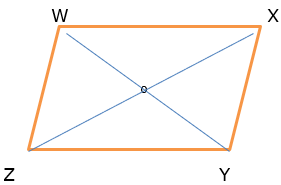Given ZX and WY are the diagonals of the parallelogram

∠ XYZ = 135° ⇒ ∠ XWZ = 135° as the opposite angels of a parallelogram are congruent.

∠YZW + ∠ XWZ = 180° as the adjacent angels of the parallelogram are supplementary.

⇒ ∠YZW = 180° - 135° = 45°

Length of OY = 5 cm then length of WY = WO + OY = 5+5 = 10 cm

(diagonals of the parallelogram bisect each other. So, O is midpoint of WY)

Question 2.

In a parallelogram ABCD, If ∠A = (3x +12)°, ∠B = (2x -32) ° then find the value of x and then find the measures of ∠C and ∠D.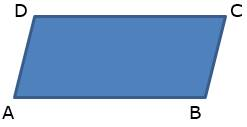∠A = (3x +12)°

∠B = (2x -32) °

∠A + ∠B = 180° (supplementary angles of the ∥gram)

(3x +12) + (2x -32) = 180

5x – 20 = 180°

5x = 200°

∴ x= 40°

∠A = (3 × 40) +12 = 120 + 12

= 132

⇒ ∠C = 132° (opposite ∠s are congruent)

Similarly, ∠B = 2× 40 – 32

= 80 - 32°

= 48°

⇒ ∠D = 48°(opposite ∠s are congruent)

Question 3.

Perimeter of a parallelogram is 150 cm. One of its sides is greater than the other side by 25 cm. Find the lengths of all sides.

perimeter of parallelogram = 150cm

Let the one side of parallelogram be x cm then

Acc. To the given condition

Other side is (x+25) cm

Perimeter of parallelogram = 2(a+b)

150 = 2( x+x+25)

150 = 2(2x+25)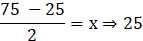One side is 25cm and the other side is 50cm.

Question 4.

If the ratio of measures of two adjacent angles of a parallelogram is 1 : 2, find the measures of all angles of the parallelogram.

Given that the ratio of measures of two adjacent angles of a parallelogram= 1 : 2

If one ∠ is x other would be 180 – x as the adjacent ∠s of a parallelogram are supplementary.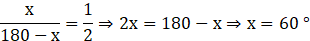Other ∠ is 120° .

The measure of all the angles are 60 °, 120 °, 60 ° and 120 ° where 60 ° and 120 ° are adjacent ∠s and 60 ° and 60 ° are congruent opposite angles.

Question 5.

Diagonals of a parallelogram intersect each other at point O. If AO = 5, BO = 12 and AB = 13 then show that 􀀍ABCD is a rhombus.

The figure is given below: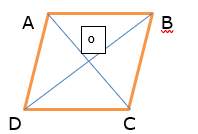Given AO =5, BO = 12 and AB = 13

In Δ AOB, AO2 + BO2 = AB2

∵ 52 + 122 = 132

252 + 1442 = 1692

so by the Pythagoras theorem

Δ AOB is right angled at ∠ AOB.

But ∠ AOB + ∠ AOD forms a linear pair so the given parallelogram is rhombus whose diagonal bisects each other at 90°.

Question 6.

In the figure 5.12, 􀀍PQRS and 􀀍ABCR are two parallelograms. If ∠P = 110° then find the measures of all angles of 􀀍ABCR.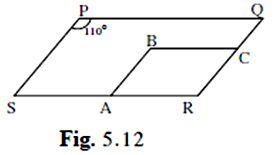given PQRS and ABCR are two ∥gram.

∠P = 110° ⇒ ∠R = 110°

(opposite ∠s of parallelogram are congruent)

Now if , ∠R = 110° ⇒ ∠ B = 110°

∠B + ∠A = 180°

(adjacent ∠s of a parallelogram are supplementary)

⇒ ∠A = 70° ⇒ ∠C = 70°

(opposite ∠s of parallelogram are congruent)

Question 7.

In figure 5.13 􀀍ABCD is a parallelogram. Point E is on the ray AB such that BE = AB then prove that line ED bisects seg BC at point F.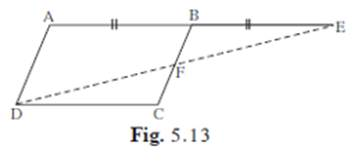Given, 􀀍ABCD is a parallelogram

And BE = AB

But AB = DC (opposite sides of the parallelogram are equal and parallel)

⇒ DC = BE

In Δ BEF and ∠DCF

∠DFC = ∠BFE (vertically opposite angles)

∠DFC = ∠ BFE (alternate ∠s on the transversal BC with AB and DC as ∥ )

And BE = AB (given)

Δ BEF ≅ ∠DCF (by AAS criterion)

⇒ BF =FC (corresponding parts of the congruent triangles)

⇒ F is mid-point of the line BC. Hence proved.

###### Practice Set 5.2
Question 1.

In figure 5.22, 􀀍ABCD is a parallelogram, P and Q are midpoints of side AB and DC respectively, then prove 􀀍APCQ is a parallelogram.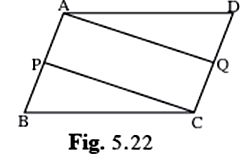Given AB ∥ to DC and AB = DC as ABCD is ∥gram.

⇒ AP ∥CQ (parts of ∥ sides are ∥) & 1/2 AB = 1/2 DC

⇒ AP = QC (P and Q are midpoint of AB and DC respectively)

⇒ AP = PB and DQ = QC

Hence APCQ is a parallelogram as the pair of opposite sides is = and ∥.

Question 2.

Using opposite angles test for parallelogram, prove that every rectangle is a parallelogram.

Opposite angle property of parallelogram says that the opposite angles of a parallelogram are congruent.

Given a rectangle which had at least one angle as 90°.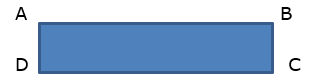If ∠ A is 90° and AD = BC (opposite sides of rectangle are ∥ and =)

AB is transversal

⇒ ∠ A + ∠B = 180 (angles on the same side of transversal is 180°)

But ∠B + ∠C is 180 (AD ∥ BC, opposite sides of rectangle)

⇒ ∠ A = ∠C = 90°

Since opposite ∠s are equal this rectangle is a parallelogram too.

Question 3.

In figure 5.23, G is the point of concurrence of medians of ΔDEF. Take point H on ray DG such that D-G-H and DG = GH, then prove that 􀀍GEHF is a parallelogram.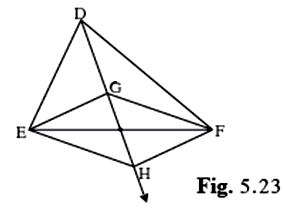Given G is the point of concurrence of medians of Δ DEF so the medians are divided in the ratio of 2:1 at the point of concurrence. Let O be the point of intersection of GH AND EF.

The figure is shown below: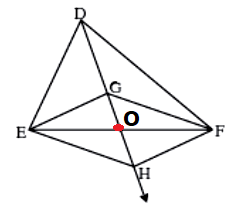⇒ DG = 2 GO

But DG = GH

⇒ 2 GO = GH

Also DO is the median for side EF.

⇒ EO = OF

Since the two diagonals bisects each other

⇒ GEHF is a ∥gram.

Question 4.

Prove that quadrilateral formed by the intersection of angle bisectors of all angles of a parallelogram is a rectangle. (Figure 5.24)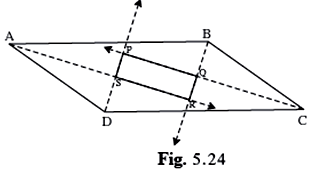Given ABCD is a parallelogram

AR bisects ∠BAD, DP bisects ∠ADC , CP bisects ∠BCD and BR bisects ∠CBA

1/2 ∠ABC = ∠RBA

∠BAR + ∠RBA = 1/2 × 180° = 90°

⇒ Δ ARB is right angled at ∠ R since its acute interior angles are complementary.

Similarly Δ DPC is right angled at ∠ P and

Also in Δ COB , ∠BOC = 90° ⇒ ∠POR = 90° (vertically opposite angles)

Similarly in ΔADS , ∠ASD = 90° = ∠PSR (vertically opposite angles)

Since vertically opposite angles are equal and measures 90° the quadrilateral is a rectangle.

Question 5.

In figure 5.25, if points P, Q, R, S are on the sides of parallelogram such that AP = BQ = CR = DS then prove that 􀀍PQRS is a parallelogram.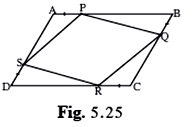Given ABCD is a parallelogram so

and DC = AB and DC ∥ AB

also AP = BQ = CR = DS

⇒ AS = CQ and PB = DR

in ΔAPS and Δ CRQ

∠ A = ∠C (opposite ∠s of a parallelogram are congruent)

AS = CQ

AP = CR

ΔAPS ≅ Δ CRQ( SAS congruence rule)

⇒ PS = RQ (c.p.c.t.)

Similarly PQ= SR

Since both the pair of opposite sides are equal

PQRS is ∥gram.

###### Practice Set 5.3
Question 1.

Diagonals of a rectangle ABCD intersect at point O. If AC = 8 cm then find BO and if ∠CAD = 35° then find ∠ACB.

The diagonals of a rectangle are congruent to each other and bisects each other at the point of intersection so since AC = 8 cm

⇒ BD = 8 cm and

O is point of intersection so DO = OB = AO = OC = 4 cm

⇒ ∠ACB = 35 °

(since AB ∥ DC and AC is transversal ∴ ∠CAD and ∠ACB are pair of alternate interior angle.)

Question 2.

In a rhombus PQRS if PQ = 7.5 then find QR. If ∠QPS = 75° then find the measure of ∠PQR and ∠SRQ.

⇒ all the sides are congruent /equal

⇒ PQ = QR = 7.5

Also ∠QPS = 75° (given)

⇒∠QPS = 75° (opposite angles are congruent)

But ∠QPS + ∠PQR = 180° (adjacent angles are supplementary)

⇒ ∠PQR = 105°

∴ ∠SRQ = 105° (opposite angles)

Question 3.

Diagonals of a square IJKL intersects at point M, Find the measures of ∠IMJ, ∠JIK and ∠LJK.

The given quadrilateral is a square

⇒ all the angles are 90°

∴ ∠JIK = 90°

Since the diagonals are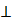to each other ∠IMJ = 90°

Since the diagonals os a square are bisectors of the angles also

∠LJK = ∠IJL = 1/2 × 90° = 45°

Question 4.

Diagonals of a rhombus are 20 cm and 21 cm respectively, then find the side of rhombus and its perimeter.

Let the diagonal AC = 20cm and BD = 21

AB2 = BO2 + AO2

AB2 = (10.5)2 + (10)2

(the diagonals of a rhombus bisect each other at 90°)

AB2 = 110.25 + 100

AB = √210.25 = 14.5cm (side of the rhombus)

Perimeter = 4a = 14. 5 × 4 = 58cm

Question 5.

State with reasons whether the following statements are ‘true’ or ‘false’.

(i) Every parallelogram is a rhombus.

(ii) Every rhombus is a rectangle.

(iii) Every rectangle is a parallelogram.

(iv) Every square is a rectangle.

(v) Every square is a rhombus.

(vi)Every parallelogram is a rectangle.

(i) False.

Explanation: Every Parallelogram cannot be the rhombus as the diagonals of a rhombus bisects each other at 90° but this is not the same with every parallelogram. Hence the statement if false.

(ii) False.

Explanation: In a rhombus all the sides are congruent but in a rectangle opposite sides are equal and parallel. Hence the given statement is false.

(iii) True.

Explanation: The statement is true as in a rectangle opposite angles and adjacent angles all are 90°. And for any quadrilateral to be parallelogram the opposites angles should be congruent.

(iv) True.

Explanation: Every square is a rectangle as all the angles of the square at 90° , diagonal bisects each other and are congruent , pair of opposite sides are equal and parallel . Hence every square is a rectangle is true statement.

(v) True.

Explanation: The statement is true as all the test of properties of a rhombus are meet by square that is diagonals are perpendicular bisects each other , opposite sides are parallel to each other and the diagonals bisects the angles.

(vi) False.

Explanation:

Every parallelogram is a rectangle is not true as rectangle has each angle of 90° measure but same is not the case with every parallelogram.

###### Practice Set 5.4
Question 1.

In 􀀍IJKL, side IJ || side KL ∠I = 108° ∠K = 53° then find the measures of ∠J and ∠L.

IJ ∥ KL and IL is transversal

∠I + ∠L = 180° (adjacent angles on the same side of the transversal)

⇒ ∠L = 180° – 108° = 72°

Now again IJ ∥ KL and JK is transversal

∠J + ∠K= 180 ° (adjacent angles on the same side of the transversal)

⇒ ∠K = 180° – 53° = 127°

Question 2.

In 􀀍ABCD, side BC || side AD, side AB ≅ sided DC If ∠A = 72° then find the measures of ∠B, and ∠D.

⇒ the quadrilateral is a parallelogram (pair of opposite sides are equal and parallel)

∠ A = 72°

⇒ ∠C = 72° (opposite angles of parallelogram are congruent)

∠B = 180° – 72° = 108° (adjacent angles of a parallelogram are supplementary)

∠D = 108° (opposite angles of parallelogram are congruent)

Question 3.

In 􀀍ABCD, side BC < side AD (Figure 5.32) side BC || side AD and if side BE ≅ side CD then prove that ∠ABC ≅ ∠DCB.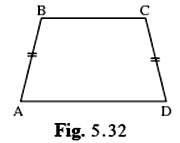The figure of the question is given below: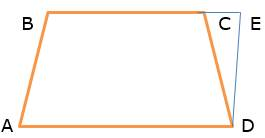Construction: we will draw a segment ∥ to BA meeting BC in E through point D.

And AB ∥ ED (construction)

⇒ AB = DE (distance between parallel lines is always same)

Hence ABDE is parallelogram

⇒ ∠ABE ≅ ∠DEC (corresponding angles on the same side of transversal)

And segBA ≅ seg DE (opposite sides of a ∥gram)

But given BA ≅ CD

So seg DE ≅ seg CD

⇒∠CED ≅ ∠DCE ( ∵ Δ CED is isosceles with CE = CD)

(Angle opposite to opposite sides are equal)

⇒ ∠ABC ≅ ∠DCB

###### Practice Set 5.5
Question 1.

In figure 5.38, points X, Y, Z are the midpoints of side AB, side BC and side AC of ΔABC respectively. AB = 5 cm, AC = 9 cm and BC = 11 cm. Find the length of XY, YZ, XZ.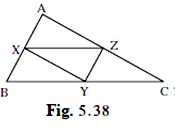Given X , Y and Z is the mid-point of AB, BC and AC.

Length of AB = 5 cm

So length of ZY = 1/2 × AB =1/2 × 5=2.5 cm (line joining mid-point of two sides of a triangle is parallel of the third side and is half of it)

Similarly, XZ = 1/2 × BC = 1/2 × 11= 5.5cm

Similarly, XY = 1/2 × AC = 1/2 × 9 = 4.5cm

Question 2.

In figure 5.39, 􀀍PQRS and 􀀍MNRL are rectangles. If point M is the midpoint of side PR then prove that,

i. SL = LR. Ii. LN = 1/2SQ.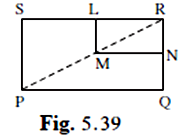The two rectangle PQRS and MNRL

In Δ PSR,

∠ PSR = ∠ MLR = 90°

∴ ML ∥ SP when SL is the transversal

M is the midpoint of PR (given)

By mid-point theorem a parallel line drawn from a mid-point of a side of a Δ meets at the Mid-point of the opposite side.

Hence L is the mid-point of SR

⇒ SL= LR

Similarly if we construct a line from L which is parallel to SR

This gives N is the midpoint of QR

Hence LN∥ SQ and L and N are mis points of SR and QR respectively

And LN = 1/2 SQ (mid-point theorem)

Question 3.

In figure 5.40, ΔABC is an equilateral triangle. Points F,D and E are midpoints of side AB, side BC, side AC respectively. Show that ΔEFD is an equilateral triangle.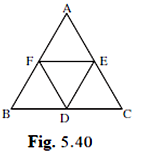Given F, D and E are mid-point of AB, BC and AC of the equilateral ΔABC ∴ AB =BC = AC

So by mid-point theorem

Line joining mid-points of two sides of a triangle is 1/2 of the parallel third side.

∴ FE = 1/2 BC =

Similarly, DE = 1/2 AB

And FD = 1/2 AC

But AB =BC = AC

⇒ 1/2 AB = 1/2 BC = 1/2 AC

⇒ DE = FD = FE

Since all the sides are equal ΔDEF is a equilateral triangle.

Question 4.

In figure 5.41, seg PD is a median of ΔPQR, Point T is the midpoint of seg PD. Produced QT intersects PR at M. Show that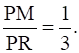[Hint : draw DN || QM.]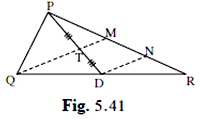PD is median so QD = DR (median divides the side opposite to vertex into equal halves)

T is mid-point of PD

⇒ PT = TD

In ΔPDN

T is mid-point and is ∥ to TM (by construction)

⇒TM is mid-point of PN

PM =MN……………….1

Similarly in ΔQMR

QM ∥ DN (construction)

D is mid –point of QR

⇒ MN = NR…………………..2

From 1 and 2

PM = MN = NR

Or PM = 1/3 PR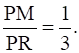hence proved

###### Problem Set 5
Question 1.

Choose the correct alternative answer and fill in the blanks.

If all pairs of adjacent sides of a quadrilateral are congruent then it is called ....
A. rectangle

B. parallelogram

C. trapezium

D. rhombus

As per the properties of a rhombus:- A rhombus is a parallelogram in which adjacent sides are equal(congruent).

Question 2.

Choose the correct alternative answer and fill in the blanks.

If the diagonal of a square is 12√2 cm then the perimeter of square is ......
A. 24 cm

B. 24√2 cm

C. 48 cm

D. 48√2 cm

Here d= 12√2 = √2 s where s is side of square

Given diagonal = 20 cm

⇒ s =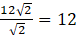Therefore, perimeter of the square is 4s = 4 x 12

= 48cm. (C)

Question 3.

Choose the correct alternative answer and fill in the blanks.

If opposite angles of a rhombus are (2x)° and (3x - 40)° then value of x is .......
A. 100°

B. 80°

C. 160°

D. 40°

As rhombus is a parallelogram with opposite angles equal

⇒ 2x = 3x -40

x= 40°

Question 4.

Adjacent sides of a rectangle are 7 cm and 24 cm. Find the length of its diagonal.

Adjacents sides are 7cm and 24 cm

In a rectangle angle between the adjacent sides is 90°

⇒ the diagonal is hypotenuse of right Δ

By pythagorus theorem

Hypotenuse2 = side2 + side2

Hypotenuse2 = 49 + 576 =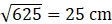length of the diagonal = 25cm

Question 5.

If diagonal of a square is 13 cm then find its side.

given Diagonal of the Square = 13cm

The angle between each side of the square is 90°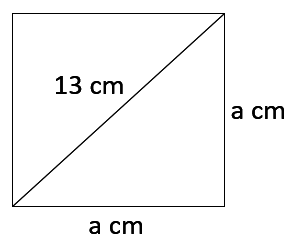Using Pythagoras theorem

Hypotenuse2 = side2 + side2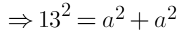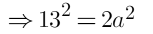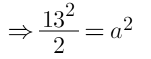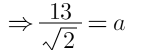Side =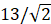cm

Question 6.

Ratio of two adjacent sides of a parallelogram is 3 : 4, and its perimeter is 112 cm. Find the length of its each side.

In a parallelogram opposite sides are equal

Let the sides of parallelogram be x and y

2x+ 2y =112 and given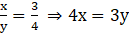⇒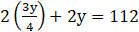⇒7y = 224

y= 32

x= 24

four sides of the parallelogram are 24cm , 32 cm, 24cm, 32cm.

Question 7.

Diagonals PR and QS of a rhombus PQRS are 20 cm and 48 cm respectively. Find the length of side PQ.

According to the properties of Rhombus diagonals of the rhombus bisects each other at 90°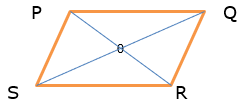In the rhombus PQRS

SO = OQ = 10 cm

PO= OR = 12cm

So in ΔPOQ

∠ POQ = 90°

⇒ PQ is hypotenuse

By Pythagoras theorem,

102 + 242 =PQ2

100 + 576 = PPQ2

676 = PQ2

26cm = PQ Ans

Question 8.

Diagonals of a rectangle PQRS are intersecting in point M. If ∠QMR = 50° then find the measure of ∠MPS.

The figure is given below: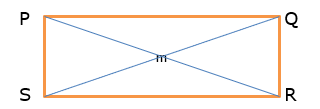Given PQRS is a rectangle

⇒ PS ∥ QR (opposite sides are equal and parallel)

QS and PR are transversal

So ∠ QMR = ∠ MPS (vertically opposite angles)

Given ∠ QMR = 50°

∴ ∠ MPS = 50°

Question 9.

In the adjacent Figure 5.42, if seg AB || seg PQ, seg AB ≅ seg PQ, seg AC || seg PR, seg AC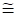seg PR then prove that, seg BC || seg QR and seg BC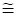seg QR.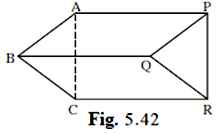Given

AB ∥ PQ

AB ≅ PQ ( or AB = PQ )

⇒ ABPQ is a parallelogram (pair of opposite sides is equal and parallel)

⇒ AP ∥ BQ and AP ≅ BQ……………..1

Similarly given,

AC ∥ PR and AC ≅ PR

⇒ACPR is a parallelogram (pair of opposite sides is equal and parallel)

⇒ AP ∥ CR and AP ≅ CR ……………………2

From 1 and 2 we get

BQ ∥ CR and BQ ≅ CR

Hence BCRQ is a parallelogram with a pair of opposite sides equal and parallel.

Hence proved.

Question 10.

In the Figure 5.43, ABCD is a trapezium. AB || DC. Points P and Q are midpoints of seg AD and seg BC respectively.

Then prove that, PQ || AB and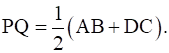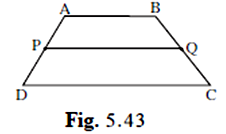Given AB ∥ DC

P and Q are mid points of AD and BC respectively.

Construction :- Join AC

The figure is given below: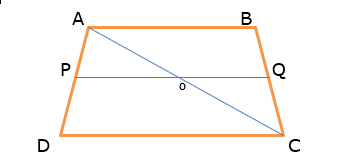P is mid point of AD and PQ is ∥ DC the part of PQ which is PO is also ∥ DC

By mid=point theorem

A line from the mid-point of a side of Δ parallel to third side, meets the other side in the mid-point

⇒ O is mid-point of AC

⇒ PO = 1/2 DC…………..1

Similarly in Δ ACB

Q id mid-point of BC and O is mid –point of AC

⇒ OQ∥ AB and OQ = 1/2 AB………………2

PO + OQ = 1/2 (DC+ AB)

PQ = 1/2 (AB +DC)

And PQ ∥ AB

Hence proved.

Question 11.

In the adjacent figure 5.44, 􀀍ABCD is a trapezium. AB || DC. Points M and N are midpoints of diagonal AC and DB respectively then prove that MN || AB.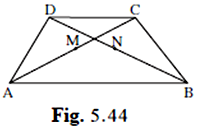Given AB ∥ DC

M is mid-point of AC and N is mid-point of DB

Given ABCD is a trapezium with AB ∥ DC

P and Q are the mid-points of the diagonals AC and BD respectively

The figure is given below: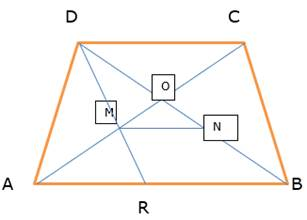To Prove:- MN ∥ AB or DC and

In ΔAB

AB || CD and AC cuts them at A and C, then

∠1 = ∠2 (alternate angles)

Again, from ΔAMR and ΔDMC,

∠1 = ∠2 (alternate angles)

AM = CM (since M is the mid=point of AC)

∠3 = ∠4 (vertically opposite angles)

From ASA congruent rule,

ΔAMR ≅ ΔDMC

Then from CPCT,

AR = CD and MR = DM

Again in ΔDRB, M and N are the mid points of the sides DR and DB,

then PQ || RB

⇒ PQ || AB

⇒ PQ || AB and CD ( ∵ AB ∥ DC)

Hence proved.

## PDF FILE TO YOUR EMAIL IMMEDIATELY PURCHASE NOTES & PAPER SOLUTION. @ Rs. 50/- each (GST extra)

SUBJECTS

HINDI ENTIRE PAPER SOLUTION

MARATHI PAPER SOLUTION
SSC MATHS I PAPER SOLUTION
SSC MATHS II PAPER SOLUTION
SSC SCIENCE I PAPER SOLUTION
SSC SCIENCE II PAPER SOLUTION
SSC ENGLISH PAPER SOLUTION
SSC & HSC ENGLISH WRITING SKILL
HSC ACCOUNTS NOTES
HSC OCM NOTES
HSC ECONOMICS NOTES
HSC SECRETARIAL PRACTICE NOTES

2019 Board Paper Solution

HSC ENGLISH SET A 2019 21st February, 2019

HSC ENGLISH SET B 2019 21st February, 2019

HSC ENGLISH SET C 2019 21st February, 2019

HSC ENGLISH SET D 2019 21st February, 2019

SECRETARIAL PRACTICE (S.P) 2019 25th February, 2019

HSC XII PHYSICS 2019 25th February, 2019

CHEMISTRY XII HSC SOLUTION 27th, February, 2019

OCM PAPER SOLUTION 2019 27th, February, 2019

HSC MATHS PAPER SOLUTION COMMERCE, 2nd March, 2019

HSC MATHS PAPER SOLUTION SCIENCE 2nd, March, 2019

SSC ENGLISH STD 10 5TH MARCH, 2019.

HSC XII ACCOUNTS 2019 6th March, 2019

HSC XII BIOLOGY 2019 6TH March, 2019

HSC XII ECONOMICS 9Th March 2019

SSC Maths I March 2019 Solution 10th Standard11th, March, 2019

SSC MATHS II MARCH 2019 SOLUTION 10TH STD.13th March, 2019

SSC SCIENCE I MARCH 2019 SOLUTION 10TH STD. 15th March, 2019.

SSC SCIENCE II MARCH 2019 SOLUTION 10TH STD. 18th March, 2019.

SSC SOCIAL SCIENCE I MARCH 2019 SOLUTION20th March, 2019

SSC SOCIAL SCIENCE II MARCH 2019 SOLUTION, 22nd March, 2019

XII CBSE - BOARD - MARCH - 2019 ENGLISH - QP + SOLUTIONS, 2nd March, 2019

HSC Maharashtra Board Papers 2020

(Std 12th English Medium)

HSC ECONOMICS MARCH 2020

HSC OCM MARCH 2020

HSC ACCOUNTS MARCH 2020

HSC S.P. MARCH 2020

HSC ENGLISH MARCH 2020

HSC HINDI MARCH 2020

HSC MARATHI MARCH 2020

HSC MATHS MARCH 2020

SSC Maharashtra Board Papers 2020

(Std 10th English Medium)

English MARCH 2020

HindI MARCH 2020

Hindi (Composite) MARCH 2020

Marathi MARCH 2020

Mathematics (Paper 1) MARCH 2020

Mathematics (Paper 2) MARCH 2020

Sanskrit MARCH 2020

Important-formula

THANKS